index: click on a letter A B C D E F G H I J K L M N O P Q R S T U V W X Y Z A to Z index index: subject areas numbers & symbols sets, logic, proofs geometry algebra trigonometry advanced algebra & pre-calculus calculus advanced topics probability & statistics real world applications multimedia entrieswww.mathwords.com about mathwords website feedback

Area of a Regular Polygon

The area of a regular polygon is given by the formula below.

area = (½)(apothem)(perimeter)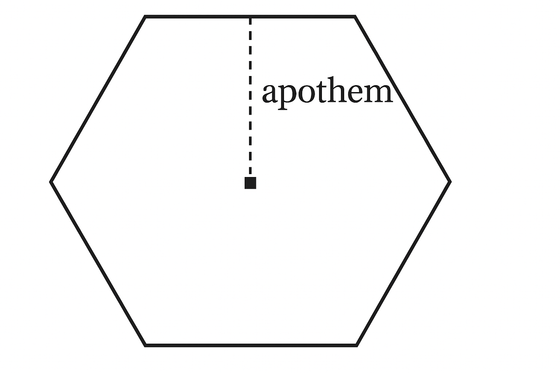Several other area formulas are also available.

 Regular Polygon Formulas n = number of sides s = length of a side r = apothem (radius of inscribed circle)    R = radius of circumcircle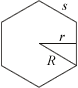Sum of interior angles = (n – 2)·180° Interior angle =Area = (½)nsr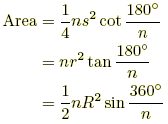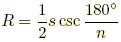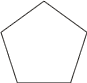Regular Pentagon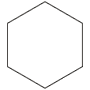Regular Hexagon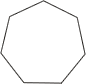Regular Heptagon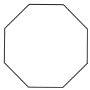Regular Octagon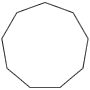Regular Nonagon